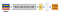# Building Machine Learning pipelines with Kubeflow

Brief introduction:

Machine Learning operations(MLOps) is a field created from applying DevOps principles to machine learning. A question that might arise is, why do we need to apply DevOps principles to machine learning? Well, to answer that, we need to understand the workflow involved in machine learning. To build a machine learning model to solve a real world problem, we need to extract data, preprocess the data, transform the data, perform data analysis, model selection, metric selection, model training, model testing, hyper parameter tuning, model validation, and then model deployment. Handling these task manually can prove to be daunting and…

# In and Out of FaceNetFaceNet Model

This article aims to give a brief introduction into the FaceNet face recognition model. Most of the terminologies and workings of the model will be explained in this article. If interested in more in-depth analysis, links to the materials referenced are provided in the link section.

Aim:

Well, the aim of facenet is quite easy to grasp, a model that can recognize and verify an individual using the face of that individual or even perform clustering i.e. finding common people based on their face.

Method:

Using deep convolutional neural networks to create Euclidean embeddings of face images in which the…

# Eigenvectors and Eigenvalues + Face Recognition = Eigen Faces

In this article, I would try to explain things as easy as possible, easy enough for you to get the intuition of it all. To give a smooth read, I would try to use as less mathematical equations as possible. If interested in the mathematics, links to the articles I referenced are provided at the end.

So, what are Eigenvectors and Eigenvalues?

To explain this I would use the eigenvector and eigenvalue equation stated below( I know I said little math but don’t worry, this is all the math needed).

A * v = v * λ … (1)

Here…

# Web scraping with Python(using BeautifulSoup)

As usual the first set of questions always go like this, what is web scraping? What is the usefulness? And how do I do it? Now, to answer the first two questions with the simplest of words, web scraping is simply the collection of specific data or information from a web site or a simple web page, to which this information or data could be used for analysis or whatever the web scraper needs such information for. Several programming languages can be used for web scraping, but as stated above we would be using the python programming language to scrape…## Kenechi Ojukwu

A software developer interested in artificial intelligence , web development and other related fields.